# Test Prep Plan - Take a practice test

Take this practice test to check your existing knowledge of the course material. We'll review your answers and create a Test Prep Plan for you based on your results.
How Test Prep Plans work
1
2Based on your results, we'll create a customized Test Prep Plan just for you!
3Study smarter
Study more effectively: skip concepts you already know and focus on what you still need to learn.

# Michigan Merit Exam: Position, Velocity & Time Chapter Exam

Exam Instructions:

Choose your answers to the questions and click 'Next' to see the next set of questions. You can skip questions if you would like and come back to them later with the yellow "Go To First Skipped Question" button. When you have completed the practice exam, a green submit button will appear. Click it to see your results. Good luck!

### Page 1

#### Question 1 1. Using the graph, calculate the displacement for the section labeled A.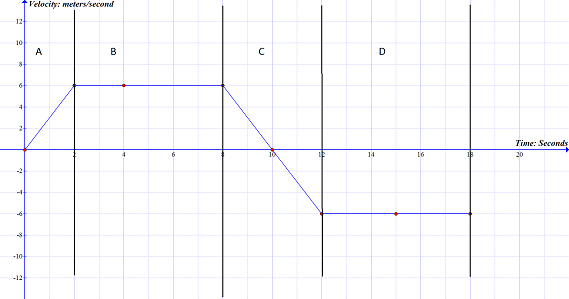#### Question 2 2. Calculate the slope of the line on the graph.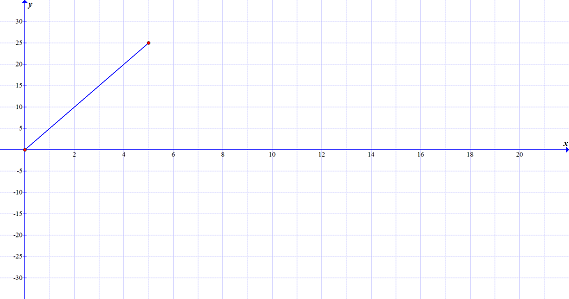#### Question 3 3. Looking at this graph, what assumption can you make about the displacement of the object over the time period indicated by the letter A?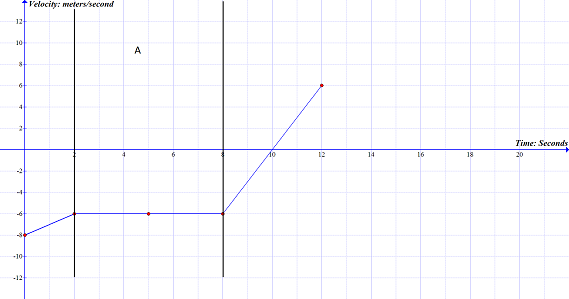#### Question 4 4. For this graph, calculate the average speed between 12 seconds and 20 seconds.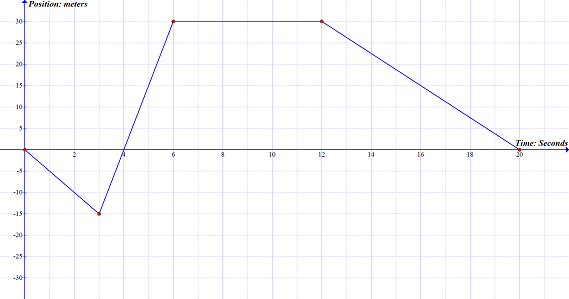#### Question 5 5. Using the graph, calculate the displacement for the section labeled D.### Page 2

#### Question 6 6. Using this graph, how long did it take for the object to move its first 10 meters?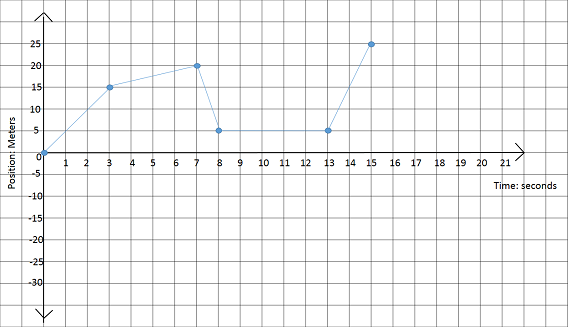#### Question 7 7. This graph represents an object that is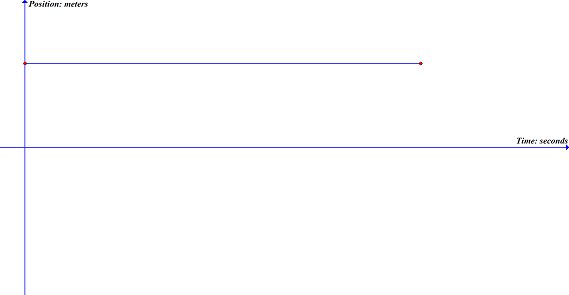### Page 3

#### Question 11 11. Using this graph, at what point is there a change in direction?#### Question 13 13. Calculate the displacement for some object in the diagram that starts at the red circle and ends at the blue triangle.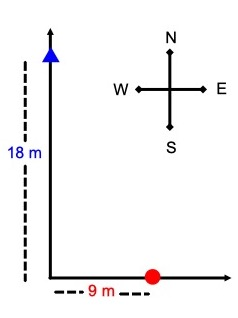#### Question 14 14. Calculate the average speed for the course, if you walked the black line in 1.7 hours.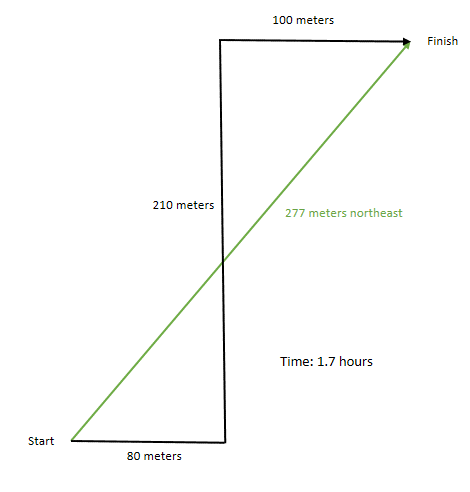### Page 4

#### Question 16 16. This graph is broken up into 5 sections, labeled A-E. On this graph, what section(s) represents no acceleration?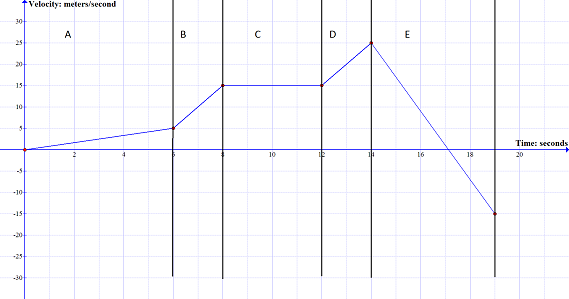#### Question 17 17. Using this graph, at what time is the object not moving?#### Question 18 18. This graph represents an object that is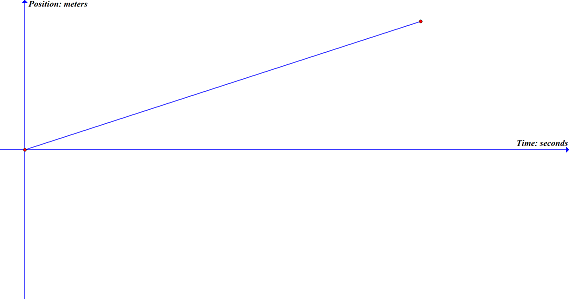#### Question 19 19. Calculate the average velocity for the course, if you walked the black line in 1.7 hours.### Page 5

#### Question 22 22. Using the graph, calculate the displacement for the section labeled B.#### Question 24 24. This graph is broken up into 5 sections, labeled A-E .On this graph, what section(s) represents negative acceleration?### Page 6

#### Question 27 27. Calculate the distance travelled by the object in the diagram.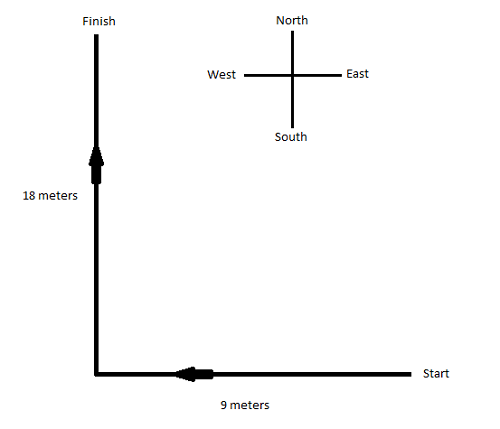#### Question 28 28. On this graph, what is the acceleration between 10 seconds and 14 seconds?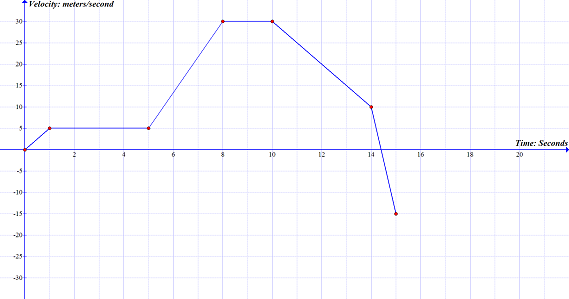#### Question 29 29. On this graph, what is the change in velocity between 5 seconds and 8 seconds?#### Question 30 30. Using this graph, what is the distance of the object from the start point at 15 seconds?#### Michigan Merit Exam: Position, Velocity & Time Chapter Exam Instructions

Choose your answers to the questions and click 'Next' to see the next set of questions. You can skip questions if you would like and come back to them later with the yellow "Go To First Skipped Question" button. When you have completed the practice exam, a green submit button will appear. Click it to see your results. Good luck!

Support